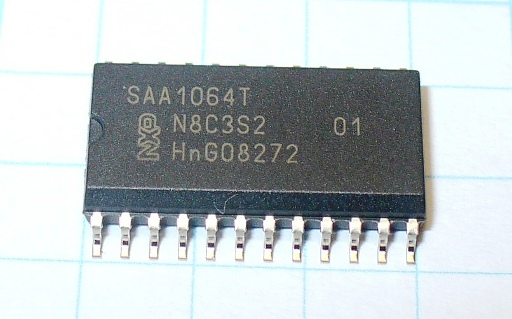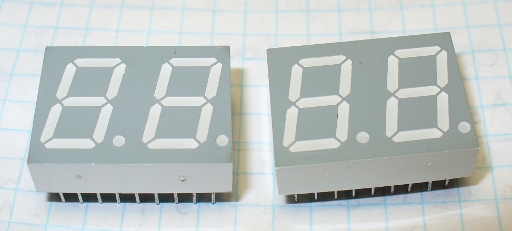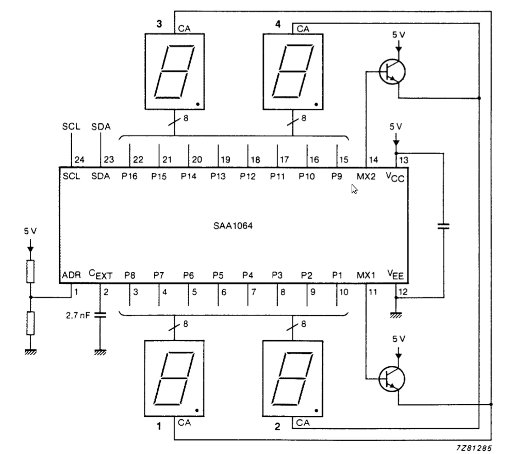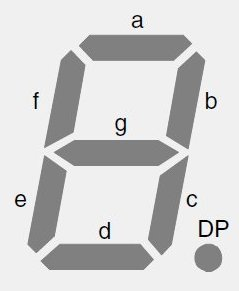# Tutorial: Arduino and the NXP SAA1064 4-digit LED display driver

In this article we investigate controlling the NXP (formerly Philips) SAA1064 4-digit LED display driver IC with Arduino and the I2C bus interface. The SAA1064 has been discontinued, however this article still gets a lot of traffic so we’ve updated it for 2019.

If you are not familiar with using the I2C bus, please read our tutorials (parts one and two) before moving on.

Furthermore as it is controlled over the I2C bus – you don’t waste any digital I/O pins on your Arduino, and you can also operate up to four SAA1064s at once (allowing 16 digits!). Finally, it has a constant-current output – keeping all the segments of your LED display at a constant brightness (which is also adjustable).  So let’s get started…

Here is an example of the SAA1064 in SOIC surface mount packaging:It measures around 15mm in length. For use in a solderless breadboard, I have soldered the IC onto a through-hole adaptor:The SAA1064 is also available in a regular through-hole DIP package. At this point, please download the data sheet (.pdf) as you will need to refer to it during the article. Next, our LED display examples. We need common-anode displays, and for this article we use two Agilent HDSP521G two-digit modules (data sheet [.pdf]) as shown below:For the uninitiated – a common anode display has all the segments’ anodes connected together, with the cathodes terminated separately. For example, our LED displays are wired as such:Notice the anodes for the left digit are pin 14, and the right digit pin 13. A device that is connected to all the cathodes (e.g. our SAA1064) will control the current flow through each element – thereby turning each segment on (and controlling the brightness) or off. Our SAA1064 is known as a current-sink as the current flows through the LED, and then sinks into the IC.

Now, let’s get it connected. There is an excellent demonstration circuit on page twelve of the data sheet that we will follow for our demonstrations:It looks pretty straight-forward, and it is. The two transistors are standard NPN-type, such as PN2222. The two transistors are used to each turn on or off a pair of digits – as the IC can only drive digits 1+3 or 2+4 together. (When presented in real life the digits are numbered 4-3-2-1).

So the pairs are alternatively turned on and off at a rapid rate, which is controlled by the capacitor between pin 2 and GND. The recommended value is 2.7 nF. At the time of writing, I didn’t have that value in stock, so chose a 3.3 nF instead. However due to the tolerance of the ceramic capacitor it was actually measured to be 2.93 nF:So close enough to 2.7 nF will be OK. The other capacitor shown between pins 12 and 13 is a standard 0.1 uF smoothing capacitor. Pin 1 on the SAA1064 is used to determine the I2C bus address – for our example we have connected it straight to GND (no resistors at all) resulting in an address of 0x70.

See the bottom page five of the data sheet for other address options. Power for the circuit can be taken from your Arduino’s 5V pin – and don’t forget to connect the circuit GND to Arduino GND. You will also use 4.7k ohm pull-up resistors on the SDA and SCL lines of the I2C bus.

The last piece of the schematic puzzle is how to connect the cathodes of the LED displays to the SAA1064. Display pins 14 and 13 are the common anodes of the digits.

The cathodes for the left-hand display module:

• LED display pins 4, 16, 15, 3, 2, 1, 18 and 17 connect to SAA1064 pins 22, 21, 20, 19, 18, 17, 16 and 15 respectively (that is, LED pin 4 to IC pin 22, etc.);
• LED display pins 9, 11, 10, 8, 6, 5, 12 and 7 also connect to SAA1064 pins 22, 21, 20, 19, 18, 17, 16 and 15 respectively.
The cathodes for the right-hand display module:
• LED display pins 4, 16, 15, 3, 2, 1, 18 and 17 connect to SAA1064 pins 3, 4, 5, 6, 7, 8, 9 and 10 respectively;
• LED display pins  9, 11, 10, 8, 6, 5, 12 and 7 also connect to SAA1064 pins 3, 4, 5, 6, 7, 8, 9 and 10 respectively.
Once your connections have been made, you could end up with spaghetti junction like this…Now it is time to consider the Arduino sketch to control out SAA1064. Each write request to the SAA1064 requires several bytes. We either send a control command (to alter some of the SAA1064 parameters such as display brightness) or a display command (actually display numbers).
For our example sketches the I2C bus address “0x70 >> 1” is stored in the byte variable saa1064. First of all, let’s look at sending commands, as this is always done first in a sketch to initiate the SAA1064 before sending it data.
As always, we send the address down the I2C bus to awaken the SAA1064 using
`Wire.beginTransmission(saa1064);`

Then the next byte is the instruction byte. If we send zero:

`Wire.write(B00000000);`

… the IC expects the next byte down the bus to be the command byte. And finally our command byte:

`Wire.write(B01000111);`

The control bits are described on page six of the data sheet. However – for four-digit operation bits 0, 1 and 2 should be 1; bit 3 should be 0; and bits 4~6 determine the amount of current allowed to flow through the LED segments. Note that they are cumulative, so if you set bits 5 and 6 to 1 – 18 mA of current will flow. We will demonstrate this in detail later on.

Next, to send actual numbers to be displayed is slightly different. Note that the digits are numbered (from left to right) 4 3 2 1. Again, we first send the address down the I2C bus to awaken the SAA1064 using
`Wire.beginTransmission(saa1064);`

Then the next byte is the instruction byte. If we send 1, the next byte of data will represent digit 1. If that follows with another byte, it will represent digit 2. And so on. So to send data to digit 1, send

`Wire.write(B00000001);`

Although sending binary helps with the explanation, you can send decimal equivalents. Next, we send a byte for each digit (from right to left).

Each bit in the byte represents a single LED element of the digit as well as the decimal point. Note how the elements are labelled (using A~G and DP) in the following image:The digit bytes describe which digit elements to turn on or off. The bytes are described as such: Bpgfedcba. (p is the decimal point).
So if you wanted to display the number 7, you would send B00000111 – as this would turn on elements a, b and c. To add the decimal point with 7 you would send B10000111.
You can also send the byte as a decimal number. So to send the digit 7 as a decimal, you would send 7 – as 00000111 in base-10 is 7.
To include the decimal point, send 135 – as 100000111 in base-10 is 135. Easy! You can also create other characters such as A~F for hexadecimal. In fact let’s do that now in the following example sketch:
```/*
Example 39.1 - NXP SAA1064 I2C LED Driver IC Demo I
Demonstrating display of digits
http://tronixstuff.com
*/
#include "Wire.h" // enable I2C bus
byte saa1064 = 0x70 >> 1; // define the I2C bus address for our SAA1064 (pin 1 to GND) ****
int digits={63, 6, 91, 79, 102, 109, 125,7, 127, 111, 119, 124, 57, 94, 121, 113};
// these are the byte representations of pins required to display each digit 0~9 then A~F
void setup()
{
Wire.begin(); // start up I2C bus
delay(500);
initDisplay();
}
void initDisplay()
// turns on dynamic mode and adjusts segment current to 12mA
{
Wire.beginTransmission(saa1064);
Wire.write(B00000000); // this is the instruction byte. Zero means the next byte is the control byte
Wire.write(B01000111); // control byte (dynamic mode on, digits 1+3 on, digits 2+4 on, 12mA segment current
Wire.endTransmission();
}
void displayDigits()
// show all digits 0~9, A~F on all digits of display
{
for (int z=0; z<16; z++)
{
Wire.beginTransmission(saa1064);
Wire.write(1); // instruction byte - first digit to control is 1 (right hand side)
Wire.write(digits[z]); // digit 1 (RHS)
Wire.write(digits[z]); // digit 2
Wire.write(digits[z]); // digit 3
Wire.write(digits[z]); // digit 4 (LHS)
Wire.endTransmission();
delay(500);
}
// now repeat but with decimal point
for (int z=0; z<16; z++)
{
Wire.beginTransmission(saa1064);
Wire.write(1); // instruction byte - first digit to control is 1 (right hand side)
Wire.write(digits[z]+128); // digit 1 (RHS)
Wire.write(digits[z]+128); // digit 2
Wire.write(digits[z]+128); // digit 3
Wire.write(digits[z]+128); // digit 4 (LHS)
Wire.endTransmission();
delay(500);
}
}
void clearDisplay()
// clears all digits
{
Wire.beginTransmission(saa1064);
Wire.write(1); // instruction byte - first digit to control is 1 (right hand side)
Wire.write(0); // digit 1 (RHS)
Wire.write(0); // digit 2
Wire.write(0); // digit 3
Wire.write(0); // digit 4 (LHS)
Wire.endTransmission();
}
void loop()
{
displayDigits();
clearDisplay();
delay(1000);
}
to GND) but 0x38 and Arduino uses 7-bit addresses- so 8-bit addresses have to
be shifted to the right one bit. Thanks to Malcolm Cox */```

In the function initDisplay() you can see an example of using the instruction then the control byte. In the function clearDisplay() you can see the simplest form of sending digits to the display – we send 0 for each digit to turn off all elements in each digit.

The bytes that define the digits 0~9 and A~F are stored in the array digits[]. For example, the digit zero is 63 in decimal, which is B00111111 in binary – which turns on elements a,b,c,d,e and f.

Finally, notice the second loop in displayDigits() – 128 is added to each digit value to turn on the decimal point. Before moving on, let’s see it in action:

Our next example revisits the instruction and control byte – we change the brightness of the digits by setting bits 4~6 in the control byte. Each level of brightness is separated into a separate function, and should be self-explanatory. Here is the sketch:

```/*
Example 39.2 - NXP SAA1064 I2C LED Driver IC Demo II
Demonstrating brightness levels via adjusting segment current
http://tronixstuff.com*/
#include "Wire.h" // enable I2C bus
byte saa1064 = 0x70 >> 1; // define the I2C bus address for our SAA1064 (pin 1 to GND)
int digits={63, 6, 91, 79, 102, 109, 125,7, 127, 111, 119, 124, 57, 94, 121, 113};
// these are the byte representations of pins required to display each digit 0~9 then A~F
void setup()
{
Wire.begin(); // start up I2C bus
delay(500);
init12ma();
}
void init3ma()
// turns on dynamic mode and adjusts segment current to 3mA
{
Wire.beginTransmission(saa1064);
Wire.write(B00000000); // this is the instruction byte. Zero means the next byte is the control byte
Wire.write(B00010111); // control byte (dynamic mode on, digits 1+3 on, digits 2+4 on, 3mA segment current
Wire.endTransmission();
}
void init6ma()
// turns on dynamic mode and adjusts segment current to 6mA
{
Wire.beginTransmission(saa1064);
Wire.write(B00000000); // this is the instruction byte. Zero means the next byte is the control byte
Wire.write(B00100111); // control byte (dynamic mode on, digits 1+3 on, digits 2+4 on, 6mA segment current
Wire.endTransmission();
}
void init9ma()
// turns on dynamic mode and adjusts segment current to 9mA
{
Wire.beginTransmission(saa1064);
Wire.write(B00000000); // this is the instruction byte. Zero means the next byte is the control byte
Wire.write(B00110111); // control byte (dynamic mode on, digits 1+3 on, digits 2+4 on, 9mA segment current
Wire.endTransmission();
}
void init12ma()
// turns on dynamic mode and adjusts segment current to 12mA
{
Wire.beginTransmission(saa1064);
Wire.write(B00000000); // this is the instruction byte. Zero means the next byte is the control byte
Wire.write(B01000111); // control byte (dynamic mode on, digits 1+3 on, digits 2+4 on, 12mA segment current
Wire.endTransmission();
}
void init18ma()
// turns on dynamic mode and adjusts segment current to 12mA
{
Wire.beginTransmission(saa1064);
Wire.write(B00000000); // this is the instruction byte. Zero means the next byte is the control byte
Wire.write(B01100111); // control byte (dynamic mode on, digits 1+3 on, digits 2+4 on, 18mA segment current
Wire.endTransmission();
}
void init21ma()
// turns on dynamic mode and adjusts segment current to 12mA
{
Wire.beginTransmission(saa1064);
Wire.write(B00000000); // this is the instruction byte. Zero means the next byte is the control byte
Wire.write(B01110111); // control byte (dynamic mode on, digits 1+3 on, digits 2+4 on, 21mA segment current
Wire.endTransmission();
}
void loop()
{
int d=250; // for delay
// first, light up all segments
Wire.beginTransmission(saa1064);
Wire.write(0); // instruction byte - Zero means the next byte is the control byte
Wire.write(B01001111); // set current and bit 3 to 1 for all segments on
Wire.endTransmission();
// now loop through the brightness levels
do
{
init3ma();
delay(d);
init6ma();
delay(d);
init9ma();
delay(d);
init12ma();
delay(d);
init18ma();
delay(d);
init21ma();
delay(d);
}
while (1>0);
}```

And again, see it in action:

For our final example, there is a function displayInteger(a,b) which can be used to easily display numbers from 0~9999 on the 4-digit display. The parameter a is the number to display, and b is the leading-zero control – zero – off, one – on.

The function does some maths on the integet to display and separates the digits for each column, then sends them to the SAA1064 in reverse order. By now you should be able to understand the following sketch:

```/*
Example 39.3 - NXP SAA1064 I2C LED Driver IC Demo III
Displaying numbers on command
https://tronixstuff.com/tutorials
*/
#include "Wire.h" // enable I2C bus
byte saa1064 = 0x70 >> 1; // define the I2C bus address for our SAA1064 (pin 1 to GND)
int digits={
63, 6, 91, 79, 102, 109, 125,7, 127, 111, 119, 124, 57, 94, 121, 113, 0};
// these are the byte representations of pins required to display each digit 0~9 then A~F, and blank digit
void setup()
{
Wire.begin(); // start up I2C bus
delay(500);
initDisplay();
}
void initDisplay()
// turns on dynamic mode and adjusts segment current to 12mA
{
Wire.beginTransmission(saa1064);
Wire.write(B00000000); // this is the instruction byte. Zero means the next byte is the control byte
Wire.write(B01000111); // control byte (dynamic mode on, digits 1+3 on, digits 2+4 on, 12mA segment current
Wire.endTransmission();
}
void clearDisplay()
{
Wire.beginTransmission(saa1064);
Wire.write(0); // blank digit 1
Wire.write(0); // blank digit 2
Wire.write(0); // blank digit 3
Wire.write(0); // blank digit 4
Wire.endTransmission();
}
void displayInteger(int num, int zero)
// displays the number 'num'
// zero = 0 - no leading zero
// zero = 1 - leading zero
{
int thousand, hundred, ten, one;
// breakdown number into columns
thousand = num/1000;
hundred = (num-(thousand*1000))/100;
ten = (num-((thousand*1000)+(hundred*100)))/10;
one = num-((thousand*1000)+(hundred*100)+(ten*10));
if (zero==1) // yes to leading zero
{
Wire.beginTransmission(saa1064);
Wire.write(1);
Wire.write(digits[one]);
Wire.write(digits[ten]);
Wire.write(digits[hundred]);
Wire.write(digits[thousand]);
Wire.endTransmission();
delay(10);
}
else
if (zero==0) // no to leading zero
{
if (thousand==0) { thousand=16; }
if (hundred==0 && num<100) { hundred=16; }
if (ten==0 && num<10) { ten=16; }
Wire.beginTransmission(saa1064);
Wire.write(1);
Wire.write(digits[one]);
Wire.write(digits[ten]);
Wire.write(digits[hundred]);
Wire.write(digits[thousand]);
Wire.endTransmission();
delay(10);
}
}
void loop()
{
for (int a=0; a<251; a++)
// display 0~250 without leading zero
{
displayInteger(a,0);
delay(20);
}
delay(1000);
clearDisplay();
delay(1000);
for (int a=0; a<1000; a++)
// display 0~999 with leading zero
{
displayInteger(a,1);
delay(5);
}
}```

And the final example in action:

So there you have it – another useful IC that can be used in conjunction with our Arduino systems to make life easier and reduce the required digital output pins.

This post brought to you by pmdway.com everything for makers and electronics enthusiasts, with free delivery worldwide.

To keep up to date with new posts at tronixstuff.com, please subscribe to the mailing list in the box on the right, or follow us on twitter @tronixstuff.